# Electronics and Communication Engineering - Electromagnetic Field Theory

46.
An isolated sphere in air has a radius equal to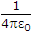meter, its capacitance is
pF
1F
4pF
1/4pF
Explanation:

C = 4p ε0 . R.

47.
Find cut off wavelength for mode TE11 in a circular waveguide having radius 2 cm. Assume K'a = 1.841
4 cm
6.82 cm
8 cm
68.2 cm
Explanation: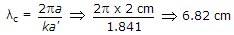.

48.
The refractive index of E layer, if angle of incidence is 30°
0.5
0.25
0
1
Explanation:

μ = sin i

49.
The sinusoidally time varying vector field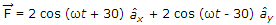is
elliptically polarized
circularly polarized
linearly polarized
unpolarized
Explanation:

60° phase, same magnitude, it form.

50.
A transmission line having 50 Ω impedance is terminated in a load of (40 + j 30) Ω. The VSWR is
j 0.033
0.8.+ j 0.6
1
2
ZC = 50 Ω, ZL = 40 + J30, r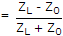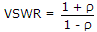.